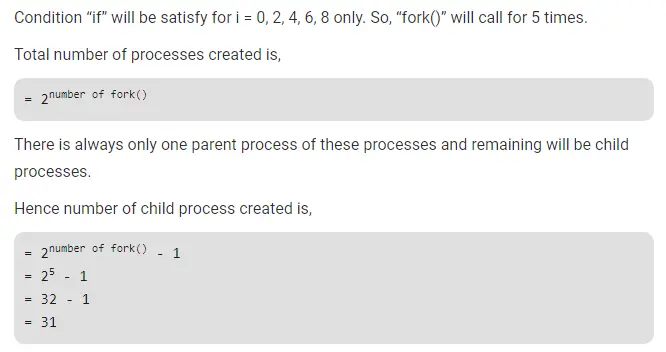# Gate CS-2019 Question Paper With Solutions

Q. 26 The following C program is executed on a Unix / Linux system:

``#include <unistd.h> ` `  ``int` `main() { ` `    ``int` `i; ` `    ``for` `(i = 0; i < 10; i++) ` `      ``if` `(i % 2 == 0) fork(); ` `    ``return` `0; ` `  ``} ``

The total number of child process created is __________ .

Note – This was Numerical Type question.

(A) 31

(B) 63

(C) 5

(D) 6